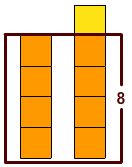Rate 0 stars Common Core
Quiz size:
Message preview:
Someone you know has shared quiz with you:

To play this quiz, click on the link below:

Hope you have a good experience with this site and recommend to your friends too.

Login to rate activities and track progress.
Login to rate activities and track progress.

Recall the doubles facts.

 1 + 1 = 2 6 + 6 = 12 2 + 2 = 4 7 + 7 = 14 3 + 3 = 6 8 + 8 = 16 4 + 4 = 8 9 + 9 = 18 5 + 5 = 10 10 + 10 = 20

Let's find the numbers whose doubles can be used to add 4 and 5.

Add 4 and 5 using doubles plus one strategy.4 + 5 = 4 + 4 + 1 = Double of 4 + 1 = 8 + 1 = 9

Now, let’s add 4 and 5 using doubles minus one strategy.4 + 5 = 5 + 5 – 1 = Double of 5 – 1 = 10 – 1 = 9

So, doubles of 4 and 5 can be used to add 4 and 5.

ds

A B C D E F G H I J K L M N O P Q R S T U V W X Y Z

### Help

##### Remember :

The smallest number is the one that comes first while counting.

##### Solution :

To arrange the given numbers in order from smallest to greatest, find the smallest number among all the given numbers.

21,27,23

21 is the smallest number.